• r语言大作业
千次阅读
2020-12-23 17:18:58

1、截取任意一个国家的新冠疫情数据。
(a) 画图疫情病例vs时间，阐述其大概趋势(5分)
(b) 分别用2种线性和2种非线性方法拟合(a)中的趋势(要求给code和拟合图)（10分）
© 对于以上4种拟合,具体阐释其拟合优劣(检查goodness of fit和对应残差图)（5分）
2、 运用R自带的iris数据库，分别用决策树和神经网络各训练一个模型，汇报模型图，含义和模型预测精度(给出完整code )(20分)
我可以代做，私信我。r语言
更多相关内容
• 数据获取 数据获取 数据获取 通过网络得到了三国志 通过网络得到了三国志 通过网络得到了三国志 通过网络得到了三国志 通过网络得到了三国志 12 全英雄武将的各项指标数据。删除掉其中日本战国和 全英雄武将的各项...R语言
• R语言.rar,R语言,实验8.docx,实验报告5.docx,第三次实验,实验教学,实验教学,新建 Microsoft Excel 工作表.xlsx,一般线性模型.docx,教学数据.xlsx,程序,多元线性回归分析案例.R,逐步回归分析.R,简单回归分析复习案例....R语言
• R.zip,预测结果.csv,聚类.Rmd,代码.R,分析报告.docx,数据预处理数据.csv,聚类.html,10079.Rproj,原始数据.csv
• 使用R语言对英雄联盟2020年中韩联赛数据进行数据预处理，分析，可视化，描述性统计，图形化展示（ggplot2），推断性统计，并且使用了帕累托方法分析数据特征。 报告中体现分析目标，解决思路，步骤，核心r函数，...
• 数据是统计局下载的，为期末大作业做准备。 数据要存成csv模式，好导入到RGui中 一、任务目的: 综合运用本学期所学统计学理论知识、R语言编程技巧和数据分析案例等内容，根据个人兴趣点，自行收集数据、整理数据、...

数据是统计局下载的，为期末大作业做准备。
数据要存成csv模式，好导入到RGui中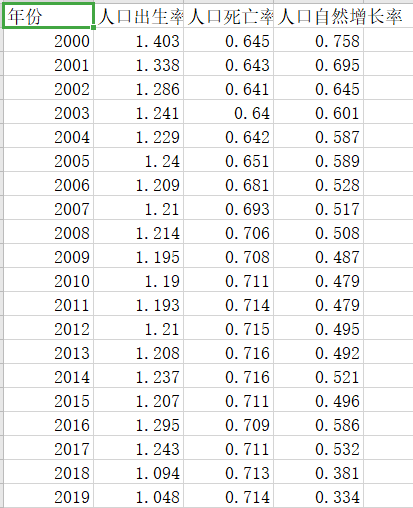年份,人口出生率,人口死亡率,人口自然增长率
2000,1.403,0.645,0.758
2001,1.338,0.643,0.695
2002,1.286,0.641,0.645
2003,1.241,0.64,0.601
2004,1.229,0.642,0.587
2005,1.24,0.651,0.589
2006,1.209,0.681,0.528
2007,1.21,0.693,0.517
2008,1.214,0.706,0.508
2009,1.195,0.708,0.487
2010,1.19,0.711,0.479
2011,1.193,0.714,0.479
2012,1.21,0.715,0.495
2013,1.208,0.716,0.492
2014,1.237,0.716,0.521
2015,1.207,0.711,0.496
2016,1.295,0.709,0.586
2017,1.243,0.711,0.532
2018,1.094,0.713,0.381
2019,1.048,0.714,0.334
（数据自行复制吧）

## 一、任务目的:

综合运用本学期所学统计学理论知识、R语言编程技巧和数据分析案例等内容，根据个人兴趣点，自行收集数据、整理数据、展示数据、分析数据，挖掘数据价值。能够正确合理使用R语言实现数据分析，并将此案例整理成数据分析报告。

## 二、任务要求:

1.数据描述性统计（平均数、标准差、中位数、偏态程度），体现数据特征，并对重要数据变量进行图表展示。
2.数据分析：明确通过数据分析要解决什么问题，运用什么分析思路、分析方法和模型，并最终得出总结性的结论或效果。
3.分析过程中，根据数据特征，解释为什么使用某某函数进行运算求解，并对运算得到的相关参数进行解读。
例如：参数估计时，数据总体服从正态分布σ未知，样本量<30,属小样本，样本均值经标准化后服从自己度为n-1的t分布，故采用t.test函数……
假设检验时，需写明具体“假设”、结论及相应代码。
建模时，需明确具体步骤，对建模参数进行解读

## 数据描述性统计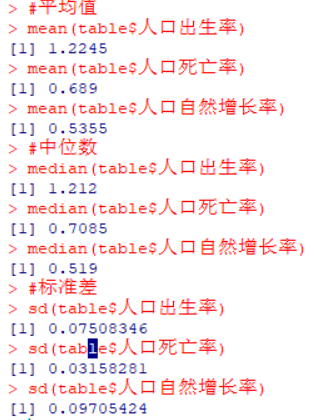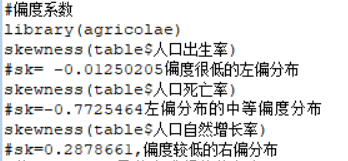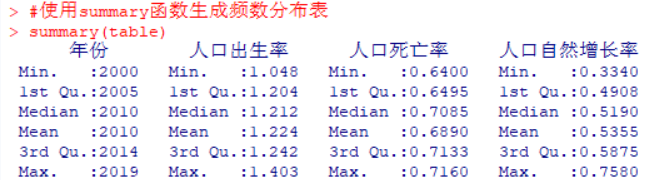## 绘制带有箱线图、轴须线和密度估计的直方图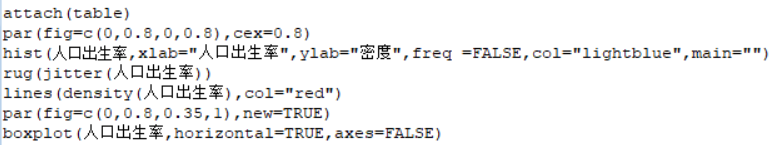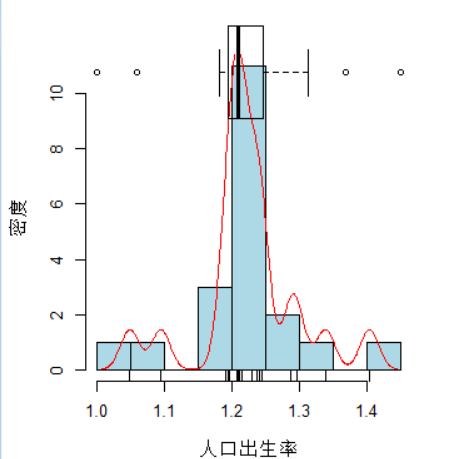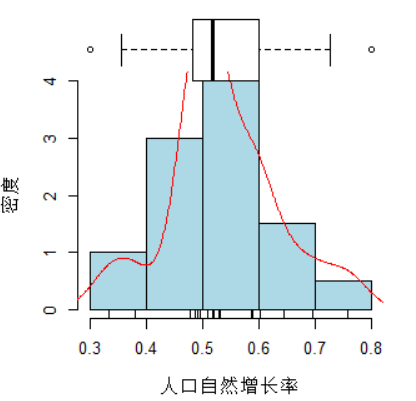## 核密度图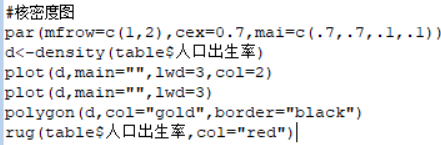人口出生率：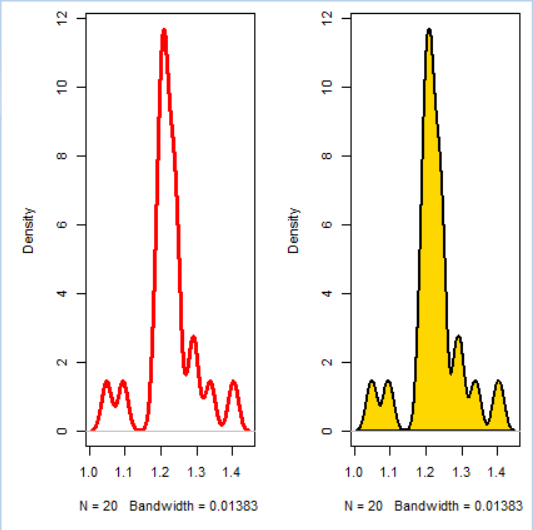人口死亡率：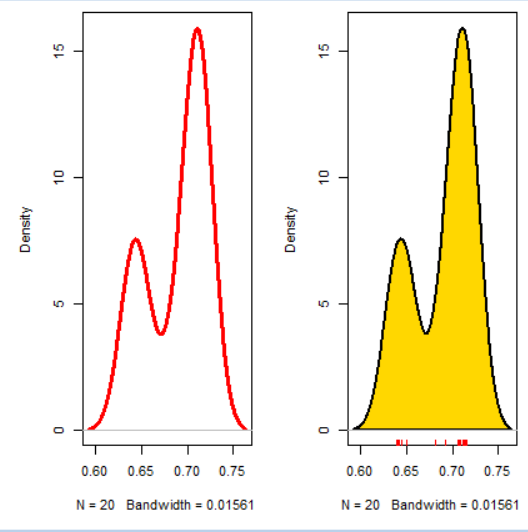人口自然增长率：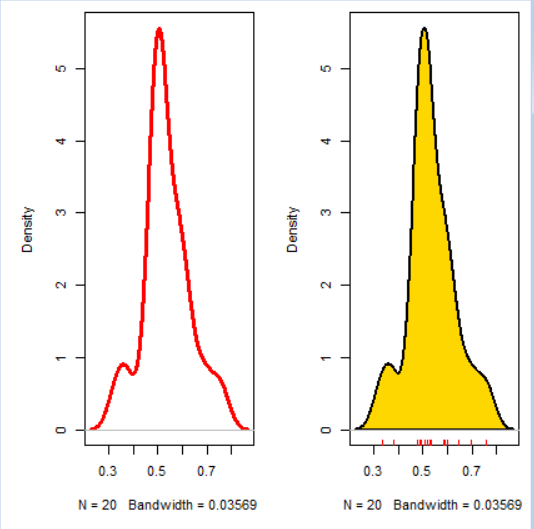## 时间序列图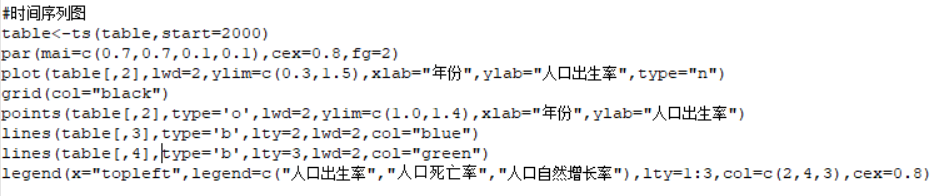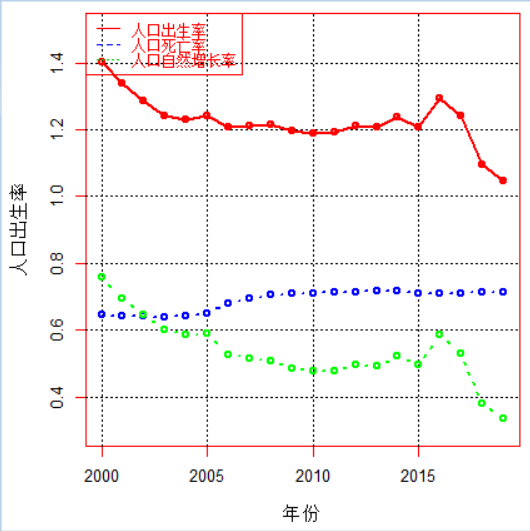## 数据分析

1. 确定变量间的关系
首先出生率和死亡率：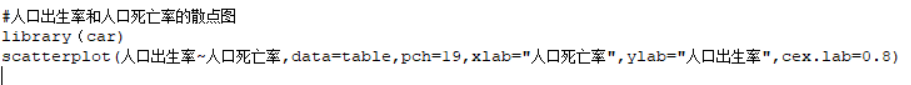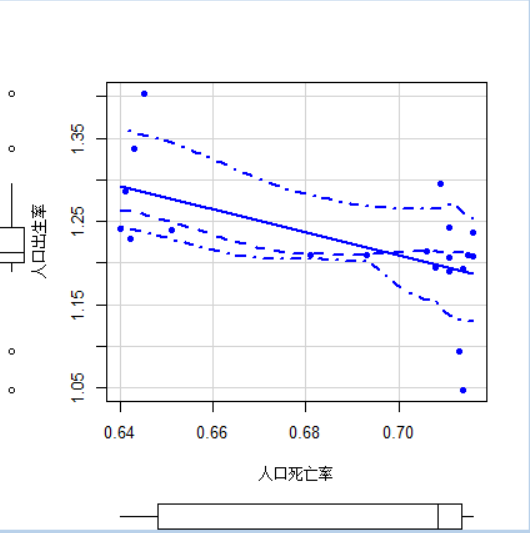人口出生率下降，人口死亡率在增长，二者的观测点分布在一条直线的周围，因而具有负线性关系。两个箱线图显示，出生率和死亡率不是对称分布。从拟合的曲线来看，有一定的线性特征，可以认为两个变量有线性关系。
其次是出生率和自然增长率
代码同上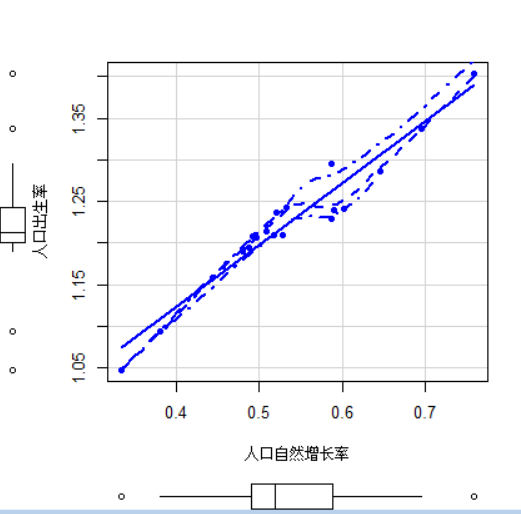人口出生率增加，人口自然增长率也在增长，二者的观测点分布在一条直线的周围，因而具有正的线性相关关系。两个箱线图显示，出生率和死亡率有一定的对称分布。从拟合的曲线来看，非线性特征不明显，显示两个变量有线性关系。
最后是死亡率和自然增长率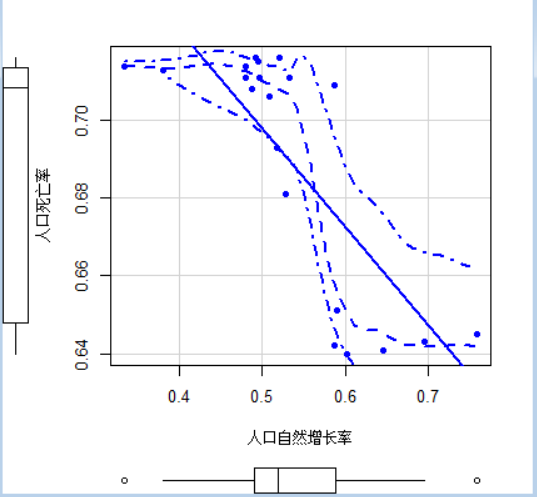人口死亡率减少，人口自然增长率在增长，二者的观测点分布在一条直线的周围，因而具有负的线性相关关系。两个箱线图显示，出生率和死亡率不是对称分布。从拟合的曲线来看，线性特征不是很明显，显示两个变量没有较强线性关系。
2. 有关于相关系数的计算与检验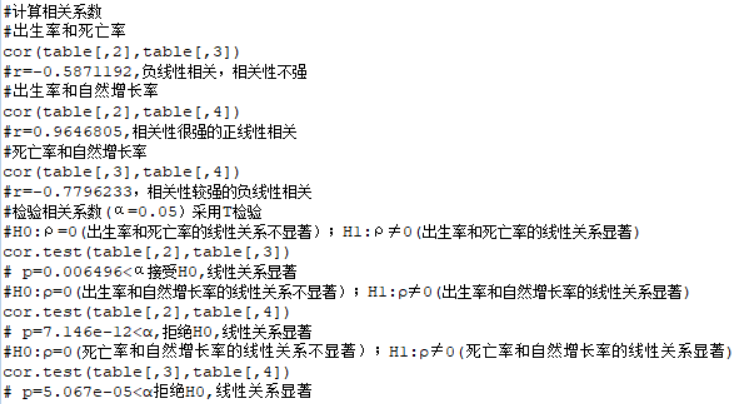3. 回归模型与回归方程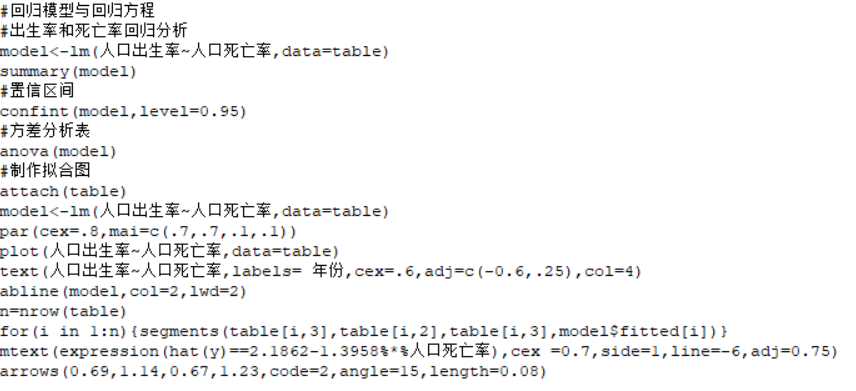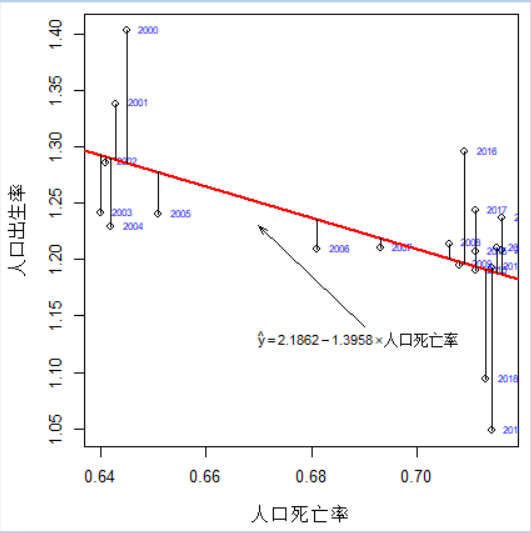arrows 函数用来在一张图表上添加箭头，只需要分别指定起始坐标和终止坐标，就可以添加箭头了，还可以通过一些属性对箭头的形状，大小进行调整.
xo, yo 指定起始点的x和y坐标，x1, y1 指定终止点的x和y坐标

arrows(x0 = 1, y0 = 1, x1 = 4, y1 = 4)


x0, y0,x1,y1 支持一次设置多个值，同时画多个箭头

arrows(x0 = c(1, 1),  y0 = c(1, 2),  x1 = c(4, 4), y1 = c(4, 5))


length ： 该参数一次只能设置一个值，默认值为0.25， 为了调整不同箭头的大小，建议分别设置

par(mfrow = c(1,3))
plot(1:5, 1:5, xlim = c(0,6), ylim = c (0,6), type = "n", main = "length = 0.1")
arrows(x0 = 1, y0 = 1, x1 = 4, y1 = 4, length = 0.1)
plot(1:5, 1:5, xlim = c(0,6), ylim = c (0,6), type = "n", main = "length = 0.5")
arrows(x0 = 1, y0 = 1, x1 = 4, y1 = 4, length = 0.5)
plot(1:5, 1:5, xlim = c(0,6), ylim = c (0,6), type = "n", main = "length = 1")
arrows(x0 = 1, y0 = 1, x1 = 4, y1 = 4, length = 1)


效果图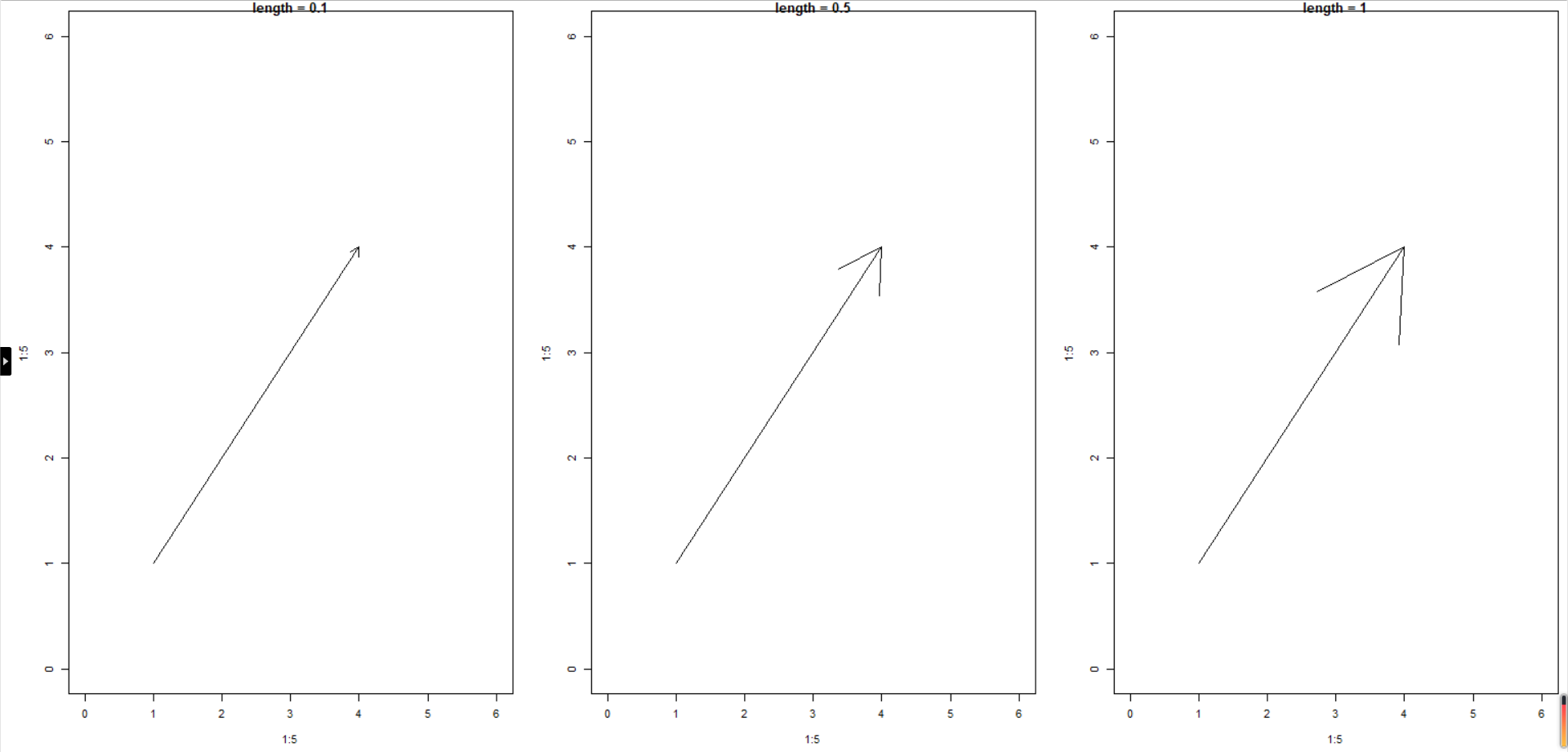code : 调整箭头的类型，一共有1,2,3,4 共四种类型，该参数一次只能设置一个值

par(mfrow = c(1,3))
plot(1:5, 1:5, xlim = c(0,6), ylim = c (0,6), type = "n", main = "code = 1")
arrows(x0 = 1, y0 = 1, x1 = 4, y1 = 4, code = 1)
plot(1:5, 1:5, xlim = c(0,6), ylim = c (0,6), type = "n", main = "code = 2")
arrows(x0 = 1, y0 = 1, x1 = 4, y1 = 4, code = 2)
plot(1:5, 1:5, xlim = c(0,6), ylim = c (0,6), type = "n", main = "code = 3")
arrows(x0 = 1, y0 = 1, x1 = 4, y1 = 4, code = 3)


效果图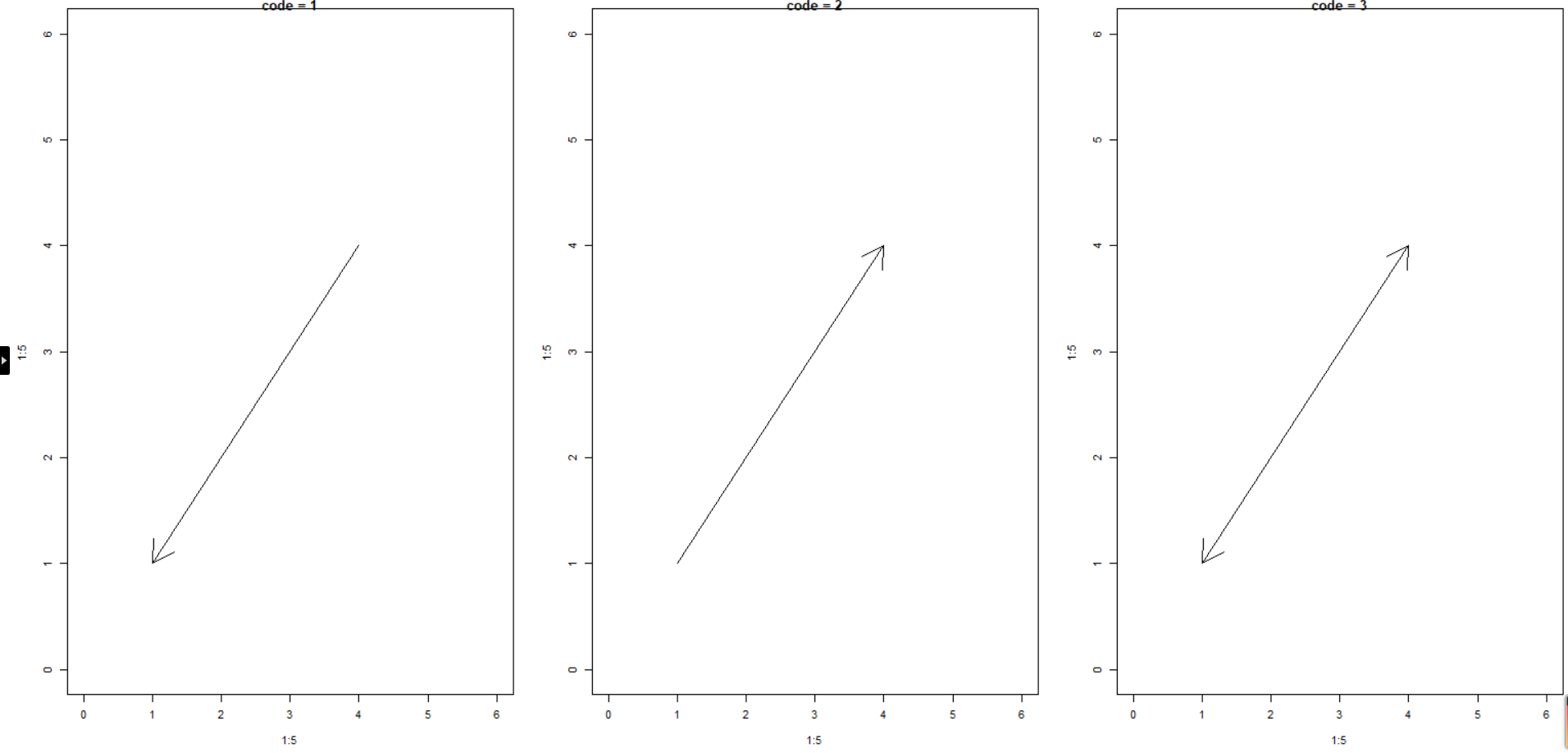angle : 设置箭头的角度，默认值是45，该参数一次只能设置一个值

par(mfrow = c(1,3))
plot(1:5, 1:5, xlim = c(0,6), ylim = c (0,6), type = "n", main = "angle = 15")
arrows(x0 = 1, y0 = 1, x1 = 4, y1 = 4, length = 0.5, angle = 15)
plot(1:5, 1:5, xlim = c(0,6), ylim = c (0,6), type = "n", main = "angle = 45")
arrows(x0 = 1, y0 = 1, x1 = 4, y1 = 4, length = 0.5, angle = 45)
plot(1:5, 1:5, xlim = c(0,6), ylim = c (0,6), type = "n", main = "angle = 60")
arrows(x0 = 1, y0 = 1, x1 = 4, y1 = 4, length = 0.5, angle = 60)


效果图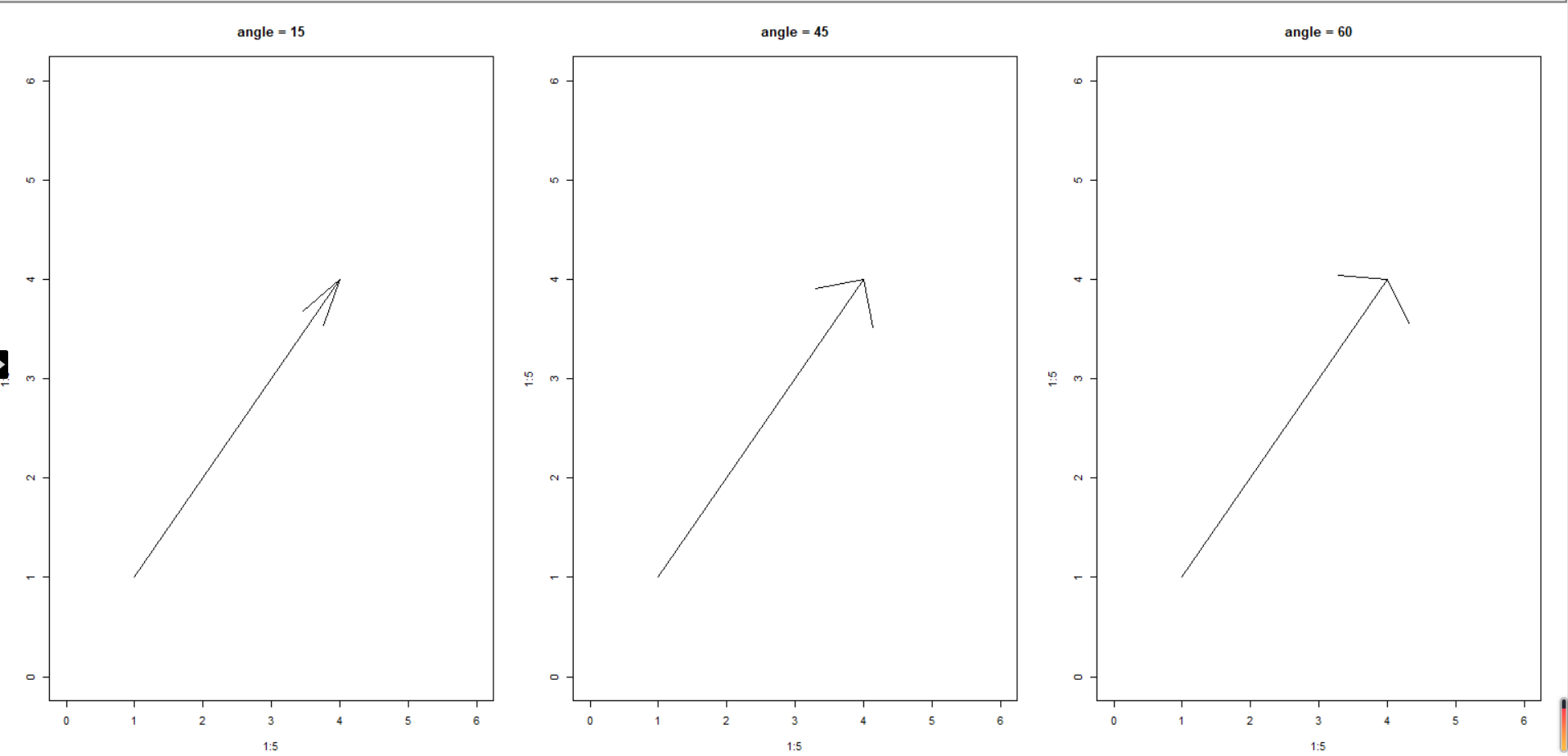除了上面的针对arrows 的特殊参数之外，也支持一些通用的参数，col , lty ,lwd 等

出生率和自然增长率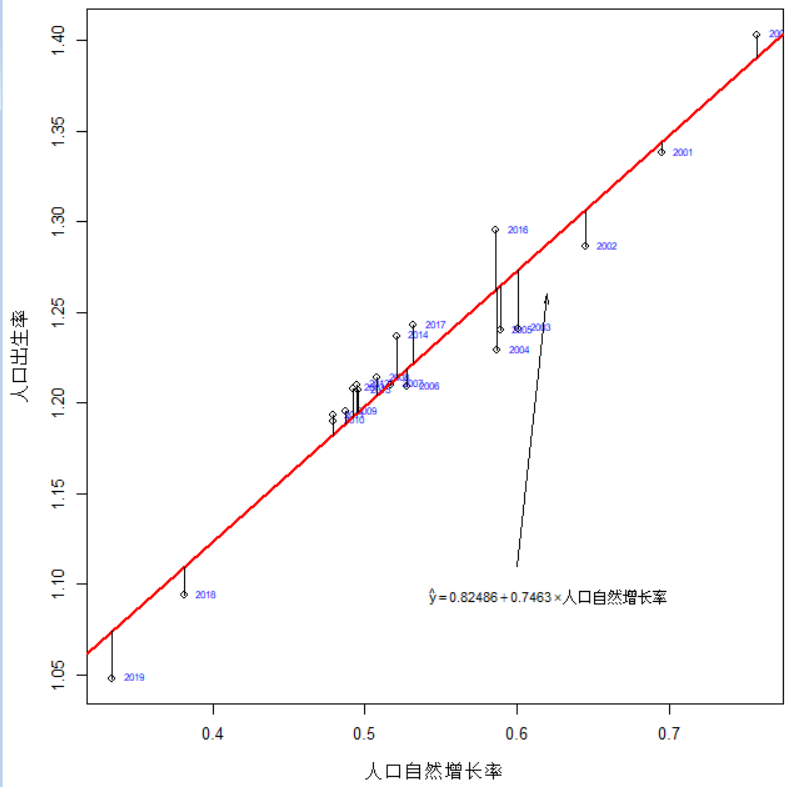死亡率和自然增长率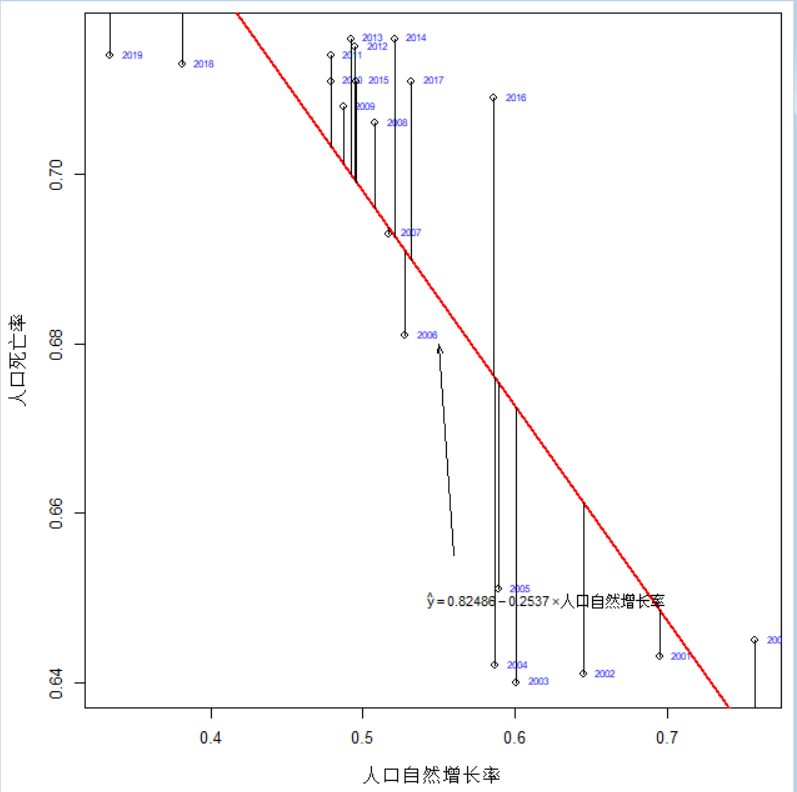## 模型的拟合优度（以出生率和死亡率为例）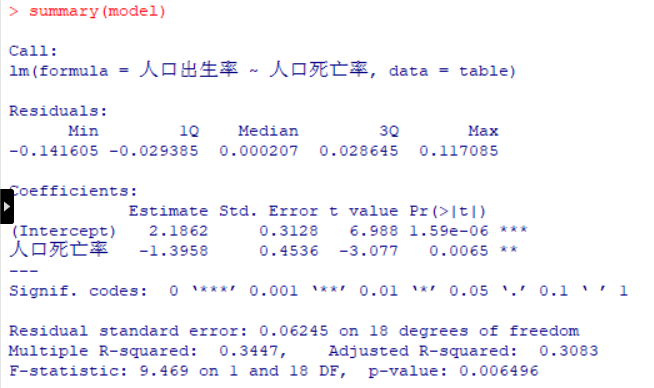出生率和死亡率的决定系数R^2=0.3447=34.47%表示在人口出生率的取值的总误差中有34.47%可以由人口出生率与死亡率之间的线性关系来解释，可见模型的拟合程度较低

## 残差的标准误

出生率和死亡率的残差标准误是0.06245，表示用死亡率来预测出生率时平均的预测误差为6.245%

## 模型显著检验——线性关系检验（F检验）

#H0：不显著；H1:显著
#F=9.469 p=0.006496<0.05拒绝原假设，线性关系显著

## 回归系数检验与推断

出生率和死亡率
H0：β1=0（自变量对因变量的影响不显著）；H1:β2≠0（显著)
t=-3.077 p=0.0065<0.05，拒绝原假设，自变量对因变量的影响显著

## 利用回归方程进行预测

#计算点预测值（pre_model）、置信区间（con_int）和预测区间（pre_int）
model<-lm(人口出生率~人口死亡率,data=table)
x0<-table$人口死亡率 pre_model<-predict(model) con_int<-predict(model,data.frame(人口死亡率=x0),interval="confidence",level=0.95) pre_int<-predict(model,data.frame(人口死亡率=x0),interval="prediction",level=0.95) pre<-data.frame(人口出生率=table$人口出生率,点预测值=pre_model,置信下限=con_int[,2],置信上限=con_int[,3],预测下限=pre_int[,2],预测上限=pre_int[,3])
pre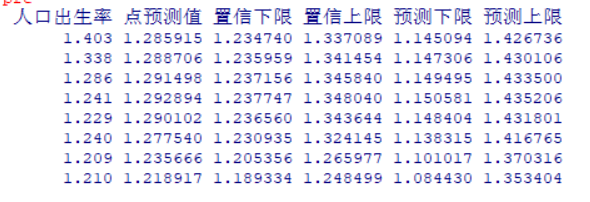# 回归模型诊断

#计算预测值（pre）、残差（res）和标准化残差（zre）（出生率，死亡率）
model<-lm(人口出生率~人口死亡率,data=table)
pre<-fitted(model)
res<-residuals(model)
zre<-model$residuals/(sqrt(deviance(model)/df.residual(model))) mysummary<-data.frame(人口出生率=table$人口出生率,点预测值=pre,残差=res,标准化残差=zre)
mysummary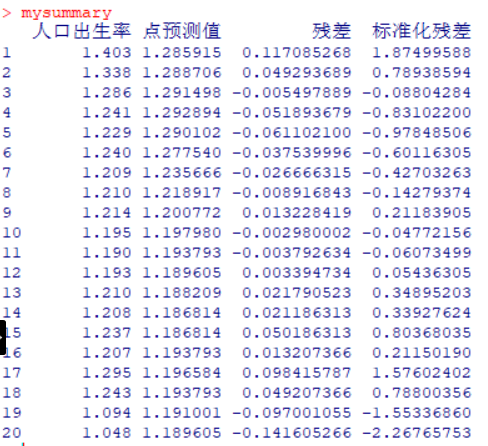# 检验线性关系

#成分残差图
model_1<-lm(人口出生率~人口死亡率,data=table)
library(car)
par(mai=c(.7,.7,.1,.1),cex=.8)
crPlots(model_1)#线性
#检验正态性
par(mfrow=c(2,2),cex=0.8,cex.main=0.7)
plot(model_1)
#检验方差齐性
library(car)
ncvTest(model_1)
#绘制散布—水平图
#检验残差独立性
library(car)
durbinWatsonTest(model_1)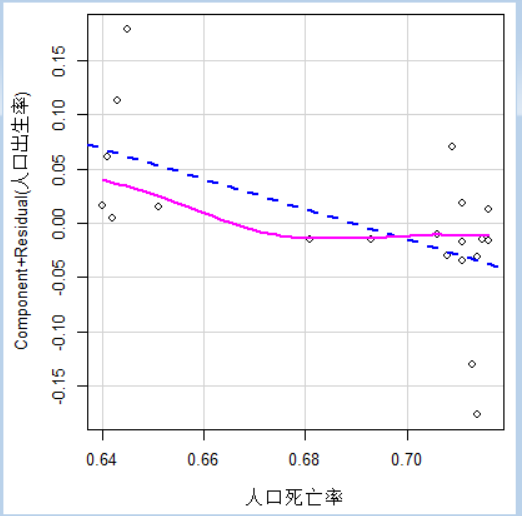残差成分图，横坐标是自变量的实际观测值，纵坐标是因变量与残差之和，从拟合的曲线可以看出，人口出生率和死亡率没有过于明显的非线性模式，说明二者的线性关系假定成立。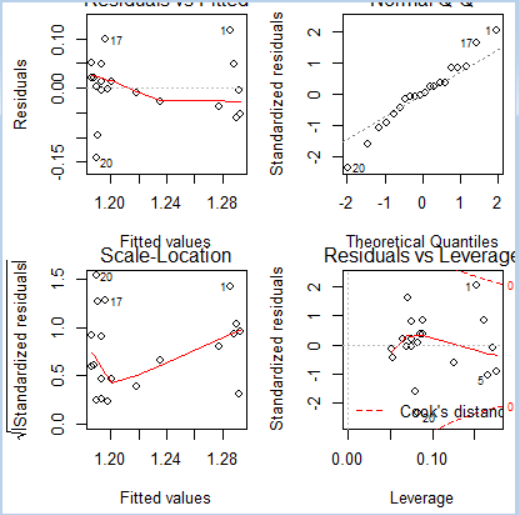右上角的图是标准化残差的正太Q-Q图，用于检验残差的正态性假定，可以看出，各个点大部分都在直线周围随机分布，没有固定模制，因此，出生率和死亡率的线性模型中，ε正态性的假定基本成立。
左上角的图是残差值与拟合值图
左下角的图是位置尺度图
右下角图是残差与杠杆图，用于鉴别样本数据是否有离群点、高杠杆点和强影响点 。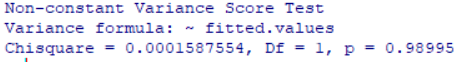方差齐性检验的原假设为误差项满足方差齐性p=0.98995接受原假设，可以认为满足方差齐性。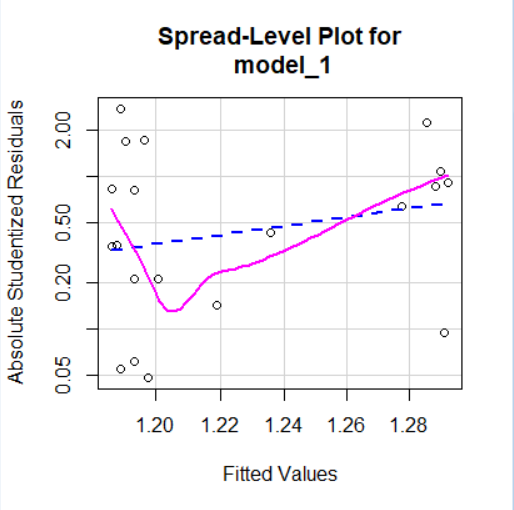该图非线性特征明显，所以不满足方差齐性的假定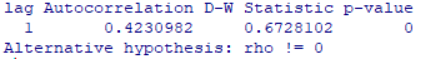原假设为残差无自相关 p=0,拒绝原假设，显示残差有自相关

展开全文r语言
•r语言 人工智能
• R语言基础期末大作业报告 需要代做加qq1975728171 环节一： 创建S3类studentS3, S4类studentS4和引用类studentRef。包含的属性分别是：姓名、学号、课程名称、学分、成绩（至少3门课程）。其中，姓名和学号分别使用...

R语言基础期末大作业报告
需要代做加qq1975728171
环节一： 创建S3类studentS3, S4类studentS4和引用类studentRef。包含的属性分别是：姓名、学号、课程名称、学分、成绩（至少3门课程）。其中，姓名和学号分别使用字符型和整数型变量，其余属性则分别是长度相等的字符型、整数型和浮点数型向量。给上述三个类设计对应的泛型函数mean()，返回出加权平均成绩。用自己的信息创建对象，调用泛型函数得出加权平均分。
此环节分值为：15分。注意：不使用自己信息创建对象不得分。

代码：

运行截图：

环节二： 编写一个函数，以自己的姓名命名，其中函数中的形式参数n是该函数的一个数值型参数，该函数用于打印1~n之间的水莲花数。调用此函数，打印出1000以内的水莲花数。（水莲花数又称阿姆斯特朗数，水仙花数是指一个整数，它的每个位上的数字的3次幂之和等于它本身。例如1^3 + 5^3+ 3^3 = 153）。此环节分值为：15分。注意：不使用自己名字作为函数名不得分。
代码：

运行截图：

环节三：以stackloss数据集中的stack.loss为因变量，分别得出对其他三个自变量的线性回归模型。创建一个2*2的画布，画出三个线性回归模型，要求每幅图中主标题为自己的姓名+因变量~自变量。针对每一个线性模型，自定义数据，封装后预测其值，并计算95%的置信区间。此环节分值为：30分。注意：不使用自己信息作为线性模型可视化标题不得分。
代码：

运行截图：

环节四：对iris数据集进行预处理，将数据集随机分为训练数据集和测试数据集，其中训练数据集占比80%，测试数据集占比20%。
对训练数据集使用朴素贝叶斯进行分类，构建分类器模型，其中分类器模型名字以自己名字命名。用测试数据集进行分类器的预测，并用混淆矩阵验证此朴素贝叶斯分类器的正确率。
对训练数据集构建决策树模型进行分类，决策树模型用自己名字+学号后三位命名，将构建出的决策树作图画出。用测试数据集进行决策树的预测并用混淆矩阵验证决策树分类的正确率。
对训练数据集构建支持向量机进行分类，构建SVM模型并用自己名字+学号后四位命名，用测试数据集进行预测并使用混淆矩阵验证构建出的SVM模型正确率。
此环节分值为:40分。注意：三个分类模型不按要求命名不得分。
代码：

运行截图：

展开全文r语言
• R语言大作业（三）：新乡市、郑州市不同学历毕业生信息统计一、实验要求二、实验准备三、数据分析（1）箱线图绘制（2）回归模型（3）数据图绘制 一、实验要求 二、实验准备 新乡市、郑州市本科生、高中毕业生信息...r语言 大数据
• R语言：上海市人口普查数据分析一、实验要求二、实验准备三、数据描述性统计四、 一、实验要求 二、实验准备 上海市人口普查数据的获取来源：上海统计局-统计年鉴 具体获取办法：手动创建表格（数据获取来源过于...r语言
• 环节二：Titanic是R的一个内置数据集，它分别以“性别”、“年龄”“舱位”“是否生存”为维度提供了泰坦尼克沉船事故中最终存活和死亡人数数据。 请绘制饼图，标题为自己的姓名，直观展现泰坦尼克号上不同舱位的...r语言
• ## R语言期末大作业

万次阅读 多人点赞 2019-07-16 12:10:52
大作业目的 针对视频游戏的销量分析，当前各游戏平台，游戏发售情况各不相同，具体分析各厂商发售的游戏以及在市场中的流行因素就具有极的现实意义，通过对其的分析能让厂商抓住市场动向从而推出受玩家欢迎...R语言
• f.rar,f,weather.csv,weatherAUS.csv,期末R语言作业.R,weather1.csv,.Rhistory,R语言期末总结报告.doc,.RData
• R语言的一些相关作业，对学习数据科学有一定帮助。r语言
• Coursera data science课程 第二次大作业R
• 我们通常使用的字符串排序是根 据字符串的首字母从小到排序，所谓逆序排序是指根据字符串的尾字母从小到排序，例 如： c("economics", "employment", "management", "finance") 的逆序排序结果是： c("finance",...R语言作业 数据科学
•r语言
•r语言
• 二、数据分析要求（使用R语言，下面是必须完成的内容）： 1.统计数据的基本统计信息，并且绘制统计图形（参照课本第2章和第3章）。 2.多元相关分析。首先绘制图形，观察自变量和因变量之间的关系，选择合适的回归...r语言
• 第三学期开放性实验课r语言作业题目.pdf第三学期开放性实验课r语言作业题目.pdf第三学期开放性实验课r语言作业题目.pdf第三学期开放性实验课r语言作业题目.pdf第三学期开放性实验课r语言作业题目.pdf
• 见习报告一份数据来自狗熊会。做了词云分析、回归分析。内置R语言代码。 版权声明：代码下载只能自己学习使用，切勿用于商业用途，违者必究。
• ## R语言课程作业

千次阅读 2020-05-14 01:16:23
1 探究感到沮丧的频率与不同年龄段之间的关系 1.1 使用的包 dplyr、ggplot2、ggmosaic、pander、tidyr 其中，ggmosaic的安装方法： devtools::install_github("haleyjeppson/ggmosaic") library(ggmosaic) ...r语言
• 成都信息工程大学，“数据统计与分析”课程作业，配套教材《数据统计分析及R语言编程》 在终端里使用 Rscript 命令执行脚本R
• 读取数据 缺失数据分析 以下程序分析数据集中缺失的数据。 统计数据集的缺失值对缺失值非常严重的无关字符变量选择直接删除，对于缺失值很少的选择插值多重插补（MI）是一种基于重复模拟处理缺失值的方法。...r语言 数据挖掘
• 针对airbnb短租数据进行深入分析，并撰写报告 报告有 3000字，个人的作业R
• 《多元统计分析与R语言作业6 针对教材P212页的表格7-5（附件文档wk_6.csv），用系统聚类和快速聚类做聚类分析。 ……今收集了8个反应城镇居民生活消费结构的指标（1996年数据见表7-5） x1x_1x1​——人均食品支出...
• R语言数据分析技术对双十一的销售数据进行统计分析并可视化，内涵数据集，程序源码及说明文档，完美应对课设及大作业...